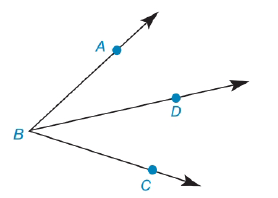Chapter 1.5, Problem 29E### Elementary Geometry for College St...

6th Edition
Daniel C. Alexander + 1 other
ISBN: 9781285195698

#### Solutions

Chapter
Section### Elementary Geometry for College St...

6th Edition
Daniel C. Alexander + 1 other
ISBN: 9781285195698
Textbook Problem
1 views

# In Exercises 27 to 30, fill in the missing reasons for each geometric proof. Given: B D → bisects ∠ A B C Prove: m ∠ A B D = 1 2 ( m ∠ A B C )Exercises 29, 30 PROOF Statements Reasons 1. B D → bisects ∠ A B C 1. ? 2. m ∠ A B D = m ∠ D B C 2. ? 3. m ∠ A B D + m ∠ D B C = m ∠ A B C 3. ? 4. m ∠ A B D + m ∠ A B D = m ∠ A B C 4. ? 5. 2 ( m ∠ A B D ) = m ∠ A B C 5. ? 6. m ∠ A B D = 1 2 ( m ∠ A B C ) 6. ?

To determine

To find:

The missing reasons for the geometric proof.

Explanation

Given:

The given table is shown below.

 PROOF Statements Reasons 1. BD→ bisects ∠ABC 1. ? 2. m∠ABD=m∠DBC 2. ? 3. m∠ABD+m∠DBC=m∠ABC 3. ? 4. m∠ABD+m∠ABD=m∠ABC 4. ? 5. 2(m∠ABD)=m∠ABC 5. ? 6. m∠ABD=12(m∠ABC) 6. ?

Property:

1. The angle bisector is defined as a line or line segment that divides an angle into two equal parts.

2. According to Angle-Addition Postulate, if point B lies in the interior of AOC then, mAOB+mBOC=mAOC.

3. According to Substitution Property of Equality, if A=B, then A can be substituted for B and B can be substituted for A in any equation.

4. The Multiplication Property of Equality states that if both the sides of an equation is multiplied by a same number then the sides of the equation remains equal.

Calculation:

The first statement of the proof is given below.

BD bisects ABC

Therefore, the first reason of the proof is Given.

Now, go from first statement to the second statement.

BD bisects ABC

Use definition of angle bisector as BD bisects ABC.

mABD=mDBC

Therefore, the second reason is definition of angle bisector.

Now, go from second statement to the third statement.

mABD=mDBC

According to Angle-Addition Postulate, the sum of the measures of ABD and DBC is equal to the measure of ABC as D is the interior of ABC.

mABD+mDBC=mABC

Therefore, the third reason for the proof is Angle-Addition Postulate.

Now, go from third statement to fourth statement.

mABD+mDBC=mABC

Use Substitution Property of Equality and substitute mABD for mDBC as mABD=mDBC.

mABD+mABD=mABC

Therefore, the fourth reason for the proof is Substitution Property of Equality.

Now, go from fourth statement to fifth statement.

mABD+mABD=mABC

Use Substitution Property of Equality and substitute 2mABD for mABD+mABD

### Still sussing out bartleby?

Check out a sample textbook solution.

See a sample solution

#### The Solution to Your Study Problems

Bartleby provides explanations to thousands of textbook problems written by our experts, many with advanced degrees!

Get Started

#### In Problems 27-30, use integration by parts to evaluate. 29.

Mathematical Applications for the Management, Life, and Social Sciences

#### Explain the difference between a matched-subjects design and a repeated-measures design.

Essentials of Statistics for The Behavioral Sciences (MindTap Course List)

#### True or False: The figure at the right is the graph of the direction field for y = (y 1)x2.

Study Guide for Stewart's Single Variable Calculus: Early Transcendentals, 8th